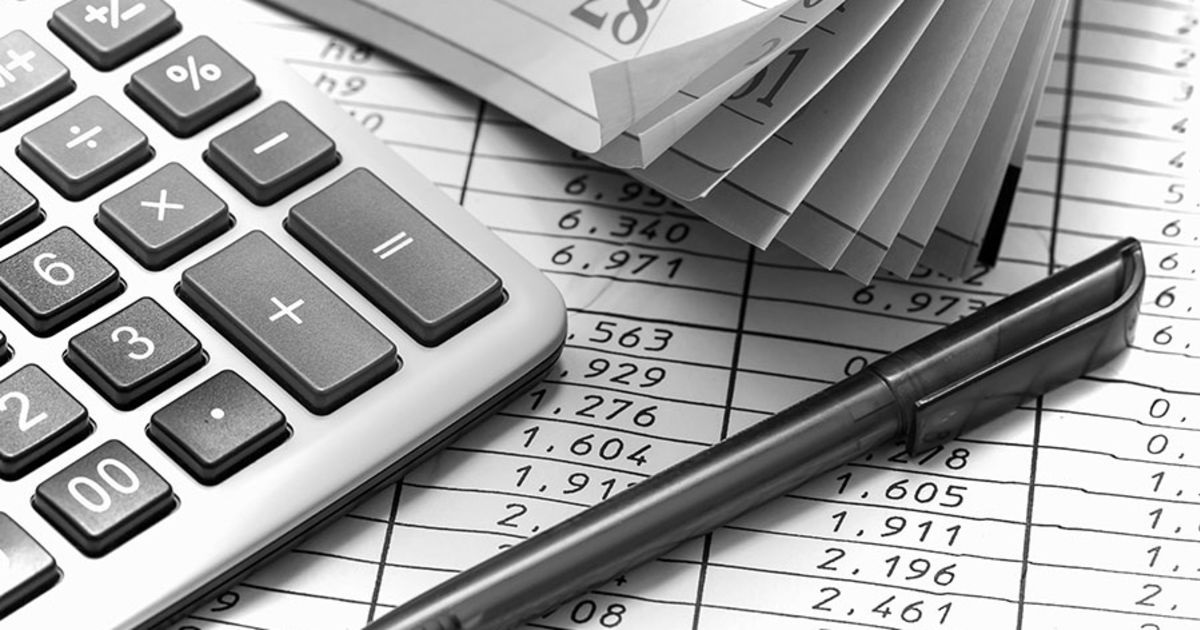# Current Yield: Ways To Determine The Bond’s Current Value

The return an investor would get if they invested at the present rate is known as the current yield. This method calculates bond returns specifically and is presented as a percentage. It would help if you grasped the fundamentals of bonds to comprehend the current yield.

Businesses can boost their cash flow by using a financial instrument called a “bond.” In essence, the investor makes a loan to the company, understanding that it would be repaid, plus interest, at a later time. A bond’s par value, also known as its face value, is in addition to the issue price (starting price) the company sets. When the bond reaches full maturity, this sum determines the bond’s final value. On the other hand, a bond has a coupon rate (or interest rate) that generates revenue from the investment at specific times. These interest payments are typically made to investors twice a year.

## The formula for Current yield

How does the Current Yield work?

By subtracting the yearly coupon from the current market price, the current yield is derived, representing the projected annual bond return.

Current Yield=             Annual Coupon

Current Bond Price

You must divide the coupon rate by the price the bond is selling for to calculate the current yield.

You need to know the cash value you are receiving from the bond due to its interest to determine the coupon amount. To utilize the result for variable A, divide the par value by the interest rate.

For example, a bond with a face value of \$2,000 and a 10% annual coupon rate would make a payment of \$200 once every year. The \$200 would be used for variable A, the coupon amount.

The sum would be regarded as the bond’s yearly cash flow. A bond will still pay back the initial amount invested in it once it has matured. However, the current yield can show you how your yearly cash flow might vary based on the market price.

## CURRENT YIELD EXAMPLE

Maria spent \$18,000 on a bond. A 7% yearly coupon rate is offered on the bond. This implies that her annual coupon payment would be \$1260. The bond is valued at \$14,500 on the open market. Based on this market rate, what would the current yield be?

Let’s dissect it to determine the significance and worth of the many factors in this issue.

Cash flow yearly: \$1260

\$14500 on the open market

Applying the values to our variables will allow us to get the current Yield:

CURRENT YIELD =    1260     = 8.69%

14500

The current yield in this situation would be 0.08689 or 8.69%.

## FINAL INSIGHT

Current yield is an analytical method used to calculate a bond’s current value based on the going market rate. It is true, this computation is quite precise. Unfortunately, it is only helpful for bonds and has little application elsewhere. However, learning them is still crucial.

For investors who wish to know their rate of return, the yield calculations (yield to call, yield to maturity, and current yield.) are highly beneficial. They can use this information to assess the investment’s merits compared to other available options.